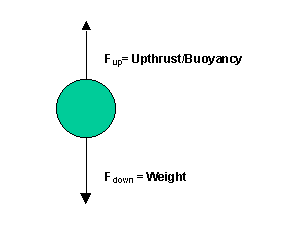# Solutions to SQA examination

### Paper I Solutions

```1. B		11. A			21. C
2. C		12. B			22. E
3. A		13. E			23. D
4. D		14. A			24. D
5. D		15. A			25. C
6. C		16. B			26. D
7. B		17. A			27. A
8. A		18. C			28. E
9. C		19. A			29. D
10.A		20. E			30. E

31.a.	Each rope exerts a force in the direction of travel.
To calculate the component of the force in the direction of travel use basic
trigonometry.

The force from each rope is:
FDirection of travel= FResultantcos20o
FDirection of travel= 150xcos20o
FDirection of travel= 140.95N

The total force from both ropes (Ftotal) can now be calculated.

Ftotal= 2x140.95N
Ftotal= 281.9N

b.	As the boat is moving at constant speed, and in a straight line, the frictional
force must be equal in magnitude to the pulling but acting in the opposite direction
to the pulling force.

32.a.b. 	Newton's first law states that: "an object will remain at rest or move with a constant
velocity in a straight line unless acted upon by an unbalanced force".
This means there are no unbalanced/resultant forces acting on the balloon, as it is at rest.

33.	To determine the type of collision the kinetic energy before the collision [Ek(before)]
and the [Ek(after)] must be considered.

Elastic collision 	=> Kinetic energy conserved.
Inelastic collision	=> Kinetic energy not conserved.

Before collision

Total [Ek(before)]=	0.036J +0J
Total [Ek(before)]=	0.036J

Total [Ek(after)]=	0.009J +0.018J
Total [Ek(after)]=	0.027J

Kinetic energy is not conserved, therefore, the collision is inelastic.

34.a.	Wave period(T) = 4x2.5ms
T  = 10x10-3s

f=1/T
f=1/10x10-3s
f=100Hz

b.	The amplitude of the waves displayed on the oscilloscope will be unchanged,
but, five complete waves will now appear on the screen.

35.a.	V = E-Ir	or	V = -rI + E
Compare this to: 	y =  mx + c

y = V
m = -r
x = I
c = E

m = (4-1)/(1-3) = 3/-2 = -1.5

=>r = -m = 1.5
=>r = 1.5W

b.	I = E/r
E is the emf of the battery, found by noting where the graph cuts the voltage axis.
E = 5.5V
=>I = 5.5/1.5
I = 3.67A

36.a.	The refractive index of paraffin(np) is found by calculating the ratio of the speed of light
in air to the speed of light in paraffin.

np = 3x108/2.1x108
np = 1.43

b.	The frequency of the light is unchanged when moving from air into paraffin.
f=4.85x1014Hz

37.
The lamp produces photons of light that have an energy that can be calculated using
the equation E=hf.Some of this energy is absorbed by the semiconductor material of
the photodiode. The absorbed energy creates electron hole pairs in the photodiode
that increases the conductivity of the photodiode.There is a reduction in the potential barrier at the pn junction and therefore a

38.	The spectrum produced by the prism is a result of refraction, whereas the grating
produces a spectrum as a result of diffraction. Furtheremore, only one spectrum of
white light is produced using a prism, whereas, several are produced using a diffraction
grating.

END OF QUESTION PAPER